×
Get Full Access to C++ For Everyone - 2 Edition - Chapter 3 - Problem R3.1
Get Full Access to C++ For Everyone - 2 Edition - Chapter 3 - Problem R3.1

×

# Find the errors in the following if statements.a.if x > 0 then cout << x;b.if (x > 0) ;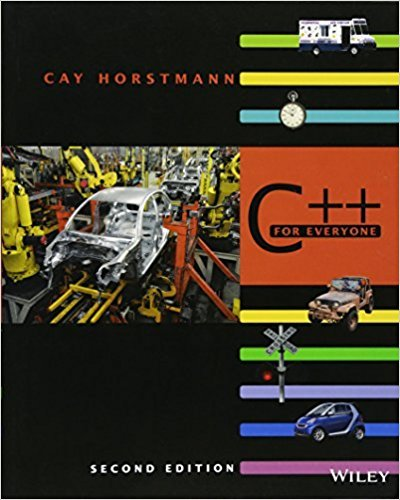ISBN: 9780470927137 356

## Solution for problem R3.1 Chapter 3

C++ for Everyone | 2nd Edition

• Textbook Solutions
• 2901 Step-by-step solutions solved by professors and subject experts
• Get 24/7 help from StudySoup virtual teaching assistantsC++ for Everyone | 2nd Edition

4 5 1 275 Reviews
31
0
Problem R3.1

Find the errors in the following if statements.a.if x > 0 then cout << x;b.if (x > 0) ; { y = 1; } else ; { y = -1; }c.if (1 + x > pow(x, sqrt(2)) { y = y + x; }d.if (x = 1) { y++; }e.cin >> x; if (cin.fail()) { y = y + x; }

Step-by-Step Solution:

R3.1 Find the errors in the following if statements.

a. if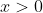then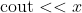;

b. if;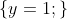else ;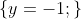c. if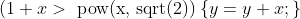d. if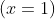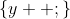e.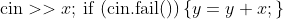Step By Step Solution

Step 1 of 5

ifthen;

Syntax error. There is an extraneous then which is not part of the C++ syntax.

We can rewrite the code as follows: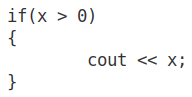Step 2 of 5

Step 3 of 5

## Discover and learn what students are asking

Statistics: Informed Decisions Using Data : Inference about Two Population Standard Deviations
?In Problems 9–14, assume that the populations are normally distributed. Test the given hypothesis. 12. s1 6 s2 at the a = 0.05 level of signi

Statistics: Informed Decisions Using Data : Inference about the Difference between Two Medians: Dependent Samples
?In Problems 3–10, use the Wilcoxon matched-pairs signedranks test to test the given hypotheses at the a = 0.05 level of significance. The dependent sa

Unlock Textbook Solution